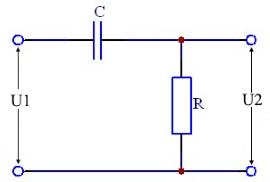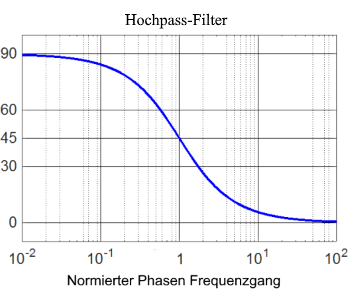# RC high pass filter

Calculator and formulas for calculating the parameters of an RC high pass filter

## RC high pass online calculator

This function calculates the properties of a high-pass filter consisting of a resistor and a capacitor. The output voltage, attenuation and phase shift are calculated for the given frequency.

RC high pass calculator

 Input Resistor mΩ Ω kΩ MΩ Capacitor F mF µF nF pF Frequency Hz kHz MHz GHz Input voltage µV mV V kV Decimal places 0 1 2 3 4 6 8 10 Result Reactance XC Output voltage U2 Attenuation dB Phase shift φ Cutoff frequency Impedance Z Voltage UC Current I Time constant τ$$\displaystyle C$$ = Capacity [F]

$$\displaystyle R$$ = Resistance [Ω]

$$\displaystyle U_1$$ = Input voltage [V]

$$\displaystyle U_2$$ = Output voltage [V]

$$\displaystyle X_C$$ = Capacitive reactance [Ω]

$$\displaystyle φ$$ = Phase angle [°]

$$\displaystyle Z$$ = Input impedance [Ω]

$$\displaystyle I$$ = Current [A]

$$\displaystyle U_C$$ = Voltage at the Capacity [V]

## Formulas for the RC high pass

### Calculate the voltage ratio

The output voltage U2 of an RC high pass is calculated according to the following formula.

$$\displaystyle U_2=U_1 ·\frac{2 · π · f · R · C} {\sqrt{1 + (2 · π · f · R · C)^2}}$$

or easier if XC is known

$$\displaystyle U_2=U_1 ·\frac{R}{\sqrt{R^2 + X_C^2}}$$
$$\displaystyle X_C=\frac{1}{2 π · f ·C}$$

### Attenuation in decibels

At the resonance frequency, the damping is 3dB. The damping can be calculated for the different frequencies using the formulas below. If the input and output voltage are known, the attenuation can easily be calculated using the following formula.

$$\displaystyle V_u=20 · lg \left(\frac{U_2}{U_1} \right)$$

If the voltages are not known, the following formula is used.

$$\displaystyle V_u=20·lg\left(\frac{2 · π · f · R · C} {\sqrt{1 + (2 · π · f · R · C)^2}}\right)$$

or simply shown

$$\displaystyle V_u=20·lg\left(\frac{ω · R · C} {\sqrt{1 + (ω · R · C)^2}}\right)$$

### Phase shift

In an RC high pass, the output voltage leads the input voltage by 0 ° - 90 °, depending on the frequency. At the resonance frequency, the phase shift is 45 °. At high frequencies it tends to 0. At low frequencies the phase shift in the direction of + 90 °. The phase shift can be calculated using the following formula.

$$\displaystyle φ=acos \left(\frac{U_2}{U_1} \right) = \left(\frac{U_a}{U_e} \right)$$
$$\displaystyle φ= arctan \left(\frac{1}{ω · R ·C}\right)$$### Cutoff frequency

At the cutof frequency fg bzw. ωg the value of the amplitude-frequency response (i.e. the magnitude of the transfer function) is 0.707, which corresponds to -3dB.

$$\displaystyle 0.707= \frac{1}{\sqrt{2}}$$

#### Cutoff frequency formulas

$$\displaystyle ω_g= \frac{1}{R ·C} ⇒$$
$$\displaystyle f_g=\frac{1}{2·π·R·C}$$

$$\displaystyle R=\frac{1}{2·π·f_g·C}$$
$$\displaystyle C=\frac{1}{2·π·f_g·R}$$

#### Impedance

$$\displaystyle Z=\sqrt{X_C^2 + R^2}$$

#### Current

$$\displaystyle I=\frac{U}{Z}$$

#### Capacitor voltage

$$\displaystyle U_C=X_C ·I$$

#### Time constant

$$\displaystyle τ=C ·R$$